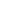# Formation of Lead-Acid Batteries and Structure of Positive and Negative Active## How the battery chemically reacts when charging and dischargingThe positive and negative poles of the battery are directly opposed to each other, but they participate in chemical reactions at the same time. When discharging, the battery is connected to the load of the external circuit, and electrons flow from the negative plate to the positive plate through the load of the external circuit, so that the potential of the positive plate drops.

When charging, it is the reverse process of the discharge reaction.The positive and negative poles of the battery are connected to the DC power supply when charging. When the power supply voltage is higher than the electromotive force E of the battery, current flows in from the positive electrode of the battery and flows out from the negative electrode of the battery, that is, electrons flow from the positive plate to the negative plate through the external circuit.

Before the negative electrode of the battery is discharged, the surface of the electrode is negatively charged, and the nearby solution is positively charged, and the two are in equilibrium. When discharging, electrons are immediately released to the external circuit. The negative charge on the electrode surface decreases, and the oxidation reaction of metal dissolution progresses slowly Me-e→Me+, which can not supplement the decrease of electrons on the electrode surface in time, and the charged state of the electrode surface changes.

This state of reduced negative charge on the surface promotes the electrons in the metal to leave the electrode, and the metal ion Me+ is transferred into the solution, accelerating the Me-e→Me+ reaction. There is always a moment to reach a new dynamic balance.

But compared with before discharge, the number of negative charges on the electrode surface is reduced, and the corresponding electrode potential becomes positive. That is, the electrochemical polarization voltage becomes higher, which seriously hinders the normal charging current. Similarly, when the positive electrode of the battery is discharged, the number of positive charges on the surface of the electrode decreases and the electrode potential becomes negative.

## How are the positive and negative voltages in the battery generatedThe reason why the current can flow in the wire is there is a difference between the high potential energy and the low potential energy in the current. This difference is called potential difference, also called voltage. In the circuit, the potential difference between any two points is called the voltage of these two points. Usually the letter U is used to represent voltage, and the unit of voltage is volts (V), referred to as volts, represented by the symbol V. High voltage can be expressed in kilovolts (kV), and low voltage can be expressed in millivolts (mV) or microvolts (μv). Voltage is the cause of current.

The voltage of the battery is also called electromotive force. There are two positive and negative electrodes in the battery. The electromotive force is the difference between the balanced electrode potentials of the two electrodes. Taking a lead-acid battery as an example, E=Ф+0-Ф-0+RT/F* In(αH2SO4/αH2O).

Among them: E—electromotive force

Ф+0—The positive electrode standard electrode potential, its value is 1.690

Ф-0—negative standard electrode potential, its value is -0.356

R-general gas constant, its value is 8.314

T-temperature, related to the temperature of the battery

F-Faraday constant, its value is 96500

αH2SO4—The activity of sulfuric acid is related to the concentration of sulfuric acid

αH2O—The activity of water is related to the concentration of sulfuric acid

It can be seen from the above formula that the standard electromotive force of a lead-acid battery is 1.690-(-0.0.356)=2.046V, so the nominal voltage of the battery is 2V. The electromotive force of lead-acid batteries is also related to temperature and sulfuric acid concentration.

When the battery is discharged, the positive reaction is: PbO2+4H++SO42-+2e-=PbSO4+2H2O

Negative reaction: Pb+SO42–2e-=PbSO4

Total reaction: PbO2+Pb+2H2SO4===2PbSO4+2H2O (the reaction to the right is discharge, and the reaction to the left is charging)

When charging, if the connection is reversed, the principle of “burning” is that in the above chemical equation, the “charging” reaction cannot proceed according to the theory, resulting in the materials in the battery cannot be recycled, and it is “burned”.